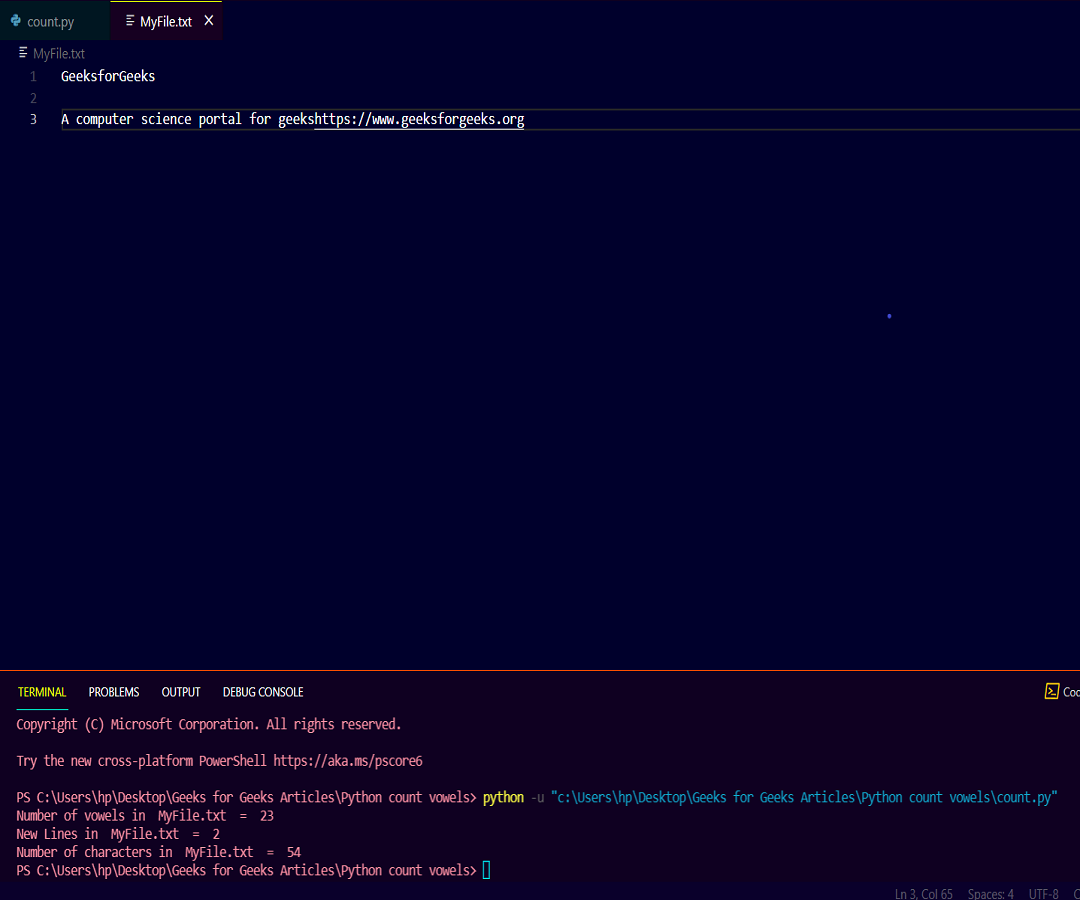Open in App
Not now

# Python Program to Count Vowels, Lines, Characters in Text File

• Difficulty Level : Basic
• Last Updated : 17 Oct, 2021

In this article, we are going to create a python program that counts vowels, lines, and a number of characters present in a particular text file.

## Approach

• We have to open the file using open() function in python.
• Then make three variables, vowel, line and character to count the number of vowels, lines, and characters respectively.
• Make a list of vowels so that we can check whether the character is a vowel or not.
• When the count hits the  ‘\n’ character we have to increase our line variable means a new line in the file.
• After that iterate over the characters of the file and count the vowels, lines, and characters.

## Python3

 `# Python program to count number of vowels,``# newlines and character in textfile`` ` `def` `counting(filename):``   ` `    ``# Opening the file in read mode``    ``txt_file ``=` `open``(filename, ``"r"``)`` ` `    ``# Initialize three variables to count number of vowels,``    ``# lines and characters respectively``    ``vowel ``=` `0``    ``line ``=` `0``    ``character ``=` `0`` ` `    ``# Make a vowels list so that we can``    ``# check whether the character is vowel or not``    ``vowels_list ``=` `[``'a'``, ``'e'``, ``'i'``, ``'o'``, ``'u'``,``                   ``'A'``, ``'E'``, ``'I'``, ``'O'``, ``'U'``]`` ` `    ``# Iterate over the characters present in file``    ``for` `alpha ``in` `txt_file.read():``       ` `        ``# Checking if the current character is vowel or not``        ``if` `alpha ``in` `vowels_list:``            ``vowel ``+``=` `1``             ` `        ``# Checking if the current character is``        ``# not vowel or new line character``        ``elif` `alpha ``not` `in` `vowels_list ``and` `alpha !``=` `"\n"``:``            ``character ``+``=` `1``             ` `        ``# Checking if the current character``        ``# is new line character or not``        ``elif` `alpha ``=``=` `"\n"``:``            ``line ``+``=` `1`` ` `    ``# Print the desired output on the console.``    ``print``(``"Number of vowels in "``, filename, ``" = "``, vowel)``    ``print``(``"New Lines in "``, filename, ``" = "``, line)``    ``print``(``"Number of characters in "``, filename, ``" = "``, character)`` ` ` ` `# Calling the function counting which gives the desired output``counting(``'Myfile.txt'``)`

Output:

```Number of vowels in  MyFile.txt  =  23
New Lines in  MyFile.txt  =  2
Number of characters in  MyFile.txt  =  54```

Text File:My Personal Notes arrow_drop_up